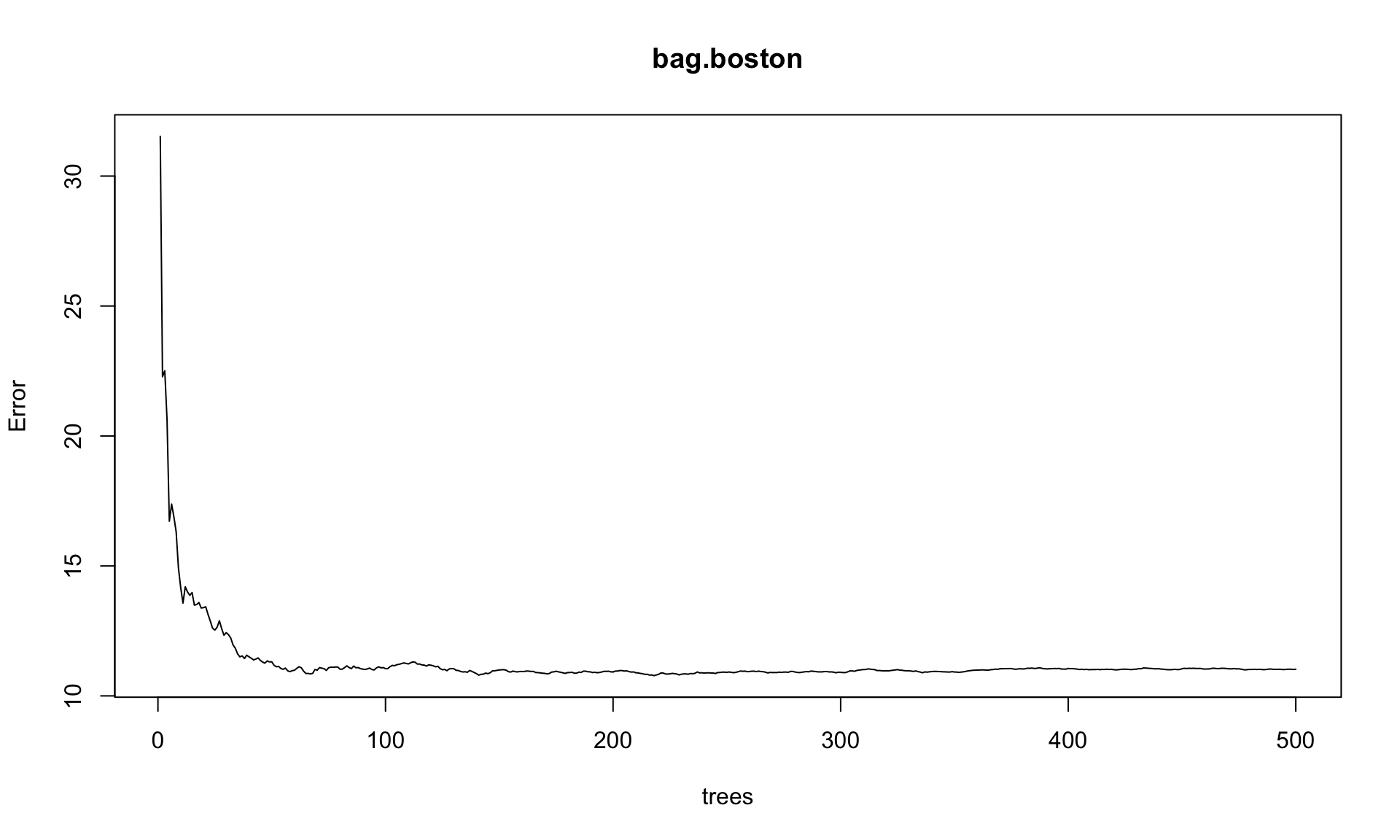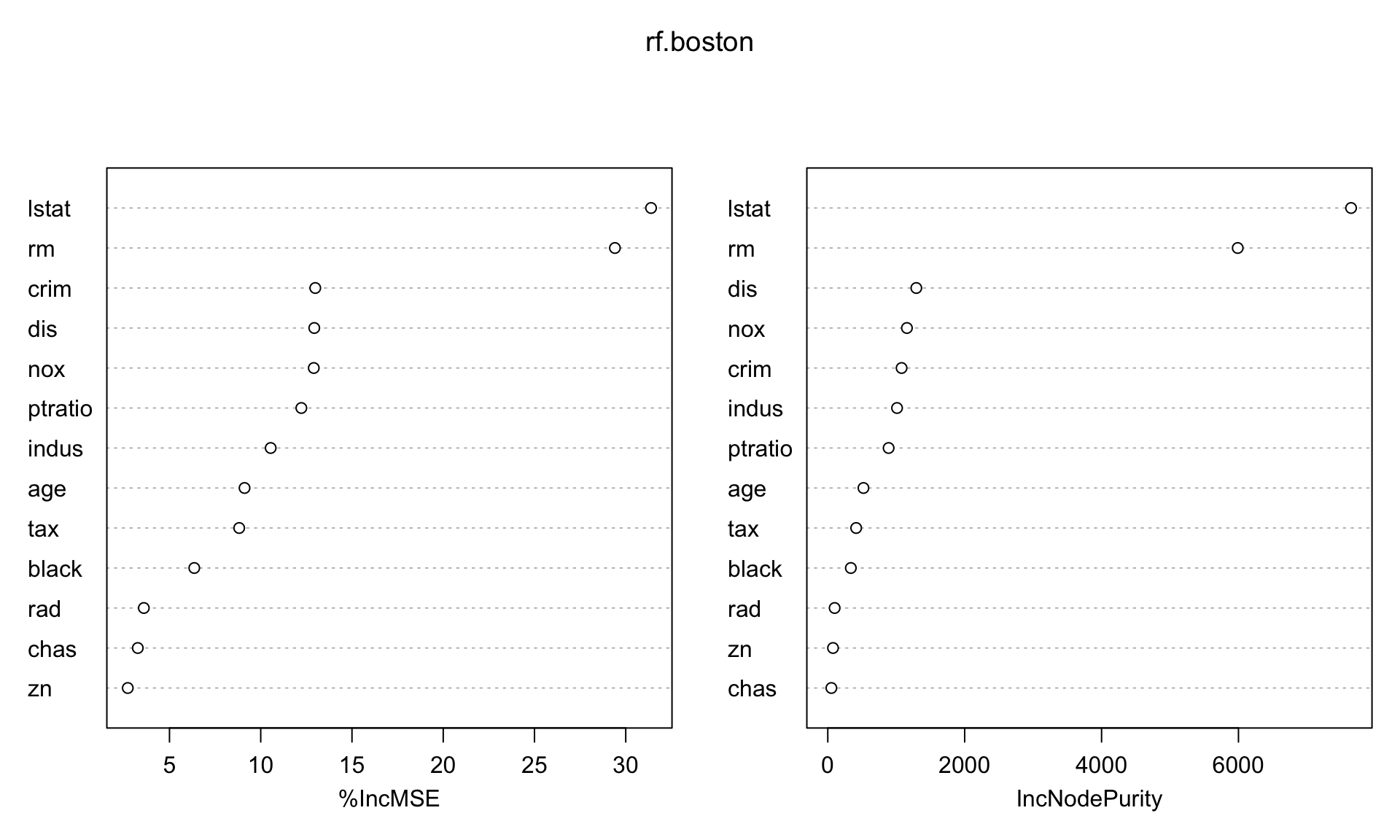``````library(ggplot2) # graphics library
library(ISLR)    # contains code and data from the textbook
library(knitr)   # contains knitting control
library(tree)    # For the tree-fitting 'tree' function
library(MASS)    # For Boston data
library(randomForest) # For random forests and bagging``````
``## randomForest 4.6-12``
``## Type rfNews() to see new features/changes/bug fixes.``
``````##
## Attaching package: 'randomForest'``````
``````## The following object is masked from 'package:ggplot2':
##
##     margin``````
``library(gbm)  # For boosting``
``## Loading required package: survival``
``## Loading required package: lattice``
``## Loading required package: splines``
``## Loading required package: parallel``
``## Loaded gbm 2.1.1``
``options(scipen = 4)  # Suppresses scientific notation``

### 1. Changing the author field and file name.

##### (b) Rename this file to “lab05_YourHameHere.Rmd”, where YourNameHere is changed to your own name.

The next portion of the lab gets you to carry out the Lab exercises in §8.3.2, 8.3.3, 10.5.1, 10.5.2 of ISL. You will want to have the textbook Lab open in front you as you go through these exercises. The ISLR Lab provides much more context and explanation for what you’re doing.

### 2. Random forests and Boosting

You will need the `Carseats` data set from the `ISLR` library in order to complete this exercise.

Please run all of the code indicated in §8.3.3-8.3.4 of ISLR, even if I don’t explicitly ask you to do so in this document.

##### (a) Run the `View()` command on the `Carseats` data to see what the data set looks like.
``#View(Carseats)``
##### The following code construst the `High` variable for the purpose of classification. Our goal will be to classify whether Carseat sales in a store are high or not.
``````High <- with(Carseats, ifelse(Sales <= 8, "No", "Yes"))
Carseats <- data.frame(Carseats, High)

# Split into training and testing
set.seed(2)
train <- sample(1:nrow(Carseats), 200)
Carseats.test <- Carseats[-train,]
High.test <- High[-train]``````
##### By setting `mtry = p` in the randomForest command, we can use the randomForest function to fit a bagged tree model. Here’s what we get.
``````# Define training set
set.seed(1)
train <- sample(1:nrow(Boston), nrow(Boston)/2)
boston.test <- Boston\$medv[-train]

set.seed(1)
bag.boston <- randomForest(medv ~ .,data=Boston, subset=train, mtry=13, importance=TRUE)
bag.boston``````
``````##
## Call:
##  randomForest(formula = medv ~ ., data = Boston, mtry = 13, importance = TRUE,      subset = train)
##                Type of random forest: regression
##                      Number of trees: 500
## No. of variables tried at each split: 13
##
##           Mean of squared residuals: 11.02509
##                     % Var explained: 86.65``````
##### (a) As always, we can use the `plot` command on a fitted model object to get some kind of plot of the model. This plot shows the Out-of-bag MSE on the y-axis. What is the plot showing on the x-axis?
``plot(bag.boston)``• This plot is showing the Out-of-bag MSE curve as a function of the number of trees (the number of bootstrapped samples). We can see that we hit a plateau around 300 trees: the error doesn’t seem to decrease much beyond that point.
##### (b) Now let’s try fitting an actual random forest. The default setting is to take `mtry = p/3` for regression and `mtry = sqrt(p)` for classification. Try fitting a random forest to the training data using `mtry = 6`. Call your fit `rf.boston`. Calculate its test set MSE.
``````rf.boston <- randomForest(medv~., data=Boston, subset=train, mtry=6, importance=TRUE)

# MSE
yhat.rf = predict(rf.boston,newdata=Boston[-train,])
mean((yhat.rf-boston.test)^2)``````
``##  11.22316``
##### (c) Run `importance` and `varImpPlot` on your random forest fit. How many plots are produced by the `varImpPlot` command? What are these plots showing?
``importance(rf.boston)``
``````##           %IncMSE IncNodePurity
## crim    12.988089    1078.43531
## zn       2.714889      76.50506
## indus   10.545100    1012.00217
## chas     3.263686      52.61111
## nox     12.906528    1156.33584
## rm      29.407391    5989.54048
## age      9.113592     521.17351
## dis     12.933480    1293.35669
## tax      8.819588     414.65202
## ptratio 12.224736     888.90254
## black    6.358499     336.69694
## lstat   31.387814    7645.22905``````
``varImpPlot(rf.boston)``• There are two plots produced by the `varImpPlot` command. Here are the details for what these plots are showing:

The first measure is computed from permuting OOB data: For each tree, the prediction error on the out-of-bag portion of the data is recorded (error rate for classification, MSE for regression). Then the same is done after permuting each predictor variable. The difference between the two are then averaged over all trees, and normalized by the standard deviation of the differences. If the standard deviation of the differences is equal to 0 for a variable, the division is not done (but the average is almost always equal to 0 in that case).

The second measure is the total decrease in node impurities from splitting on the variable, averaged over all trees. For classification, the node impurity is measured by the Gini index. For regression, it is measured by residual sum of squares.

##### (d) Use the `partialPlot` command to get partial dependence plots for the 2 most important variables according to the %IncMSE importance. Describe what you see.
``partialPlot(rf.boston, Boston, x.var = "lstat")``